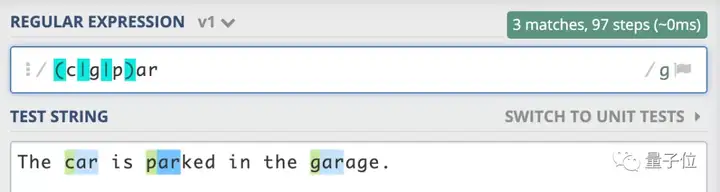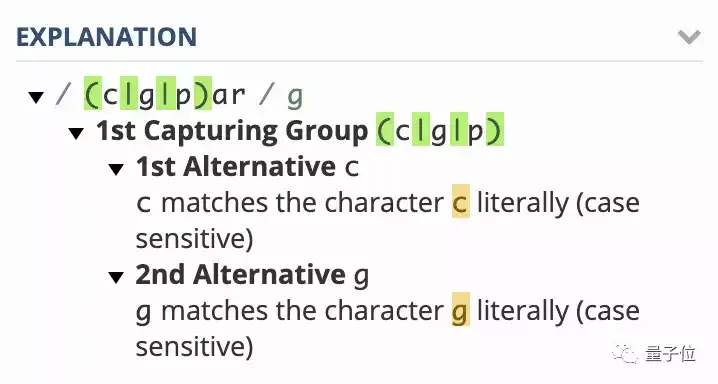## 引言

Regular expression is a group of characters or symbols which is used to find a specific pattern from a text.## regex101平台介绍是不是比枯燥的文字解释要生动很多呢？我们可以在平台上输入一系列字符串，以达到更好的练习效果，也能更好地理解正则表达式每一个规则的含义。下面我们就从基本匹配规则开始，一起来研究正则表达式吧！

## Regex的基本匹配规则

```"the" => The fat cat sat on the mat.
```

```"The" => The fat cat sat on the mat.
```

## Regex中的元字符

*正则表达式主要依赖于元字符. * 元字符不代表他们本身的字面意思, 他们都有特殊的含义. 一些元字符写在方括号中的时候有一些特殊的意思. 以下是一些元字符的介绍:

. 句号匹配任意单个字符，除了换行符.
[ ] 字符种类. 匹配方括号内的任意字符.
[^ ] 否定的字符种类. 匹配除了方括号里的任意字符
* 匹配>=0个重复的在*号之前的字符.
+ 匹配>=1个重复的+号前的字符.
? 标记?之前的字符为可选.
{n,m} 匹配num个大括号之前的字符 (n <= num <= m).
(xyz) 字符集,匹配与 xyz 完全相等的字符串.
| 或运算符,匹配符号前或后的字符.
\ 转义字符,用于匹配一些保留的字符 `[ ] ( ) { } . * + ? ^ \$ \
^ 从开始行开始匹配.开始标记
\$ 从末端开始匹配.结束标记

### 点运算符`.`

`.`匹配任意单个字符, 但不匹配换行符.

```".ar" => The car parked in the garage.
```

### 字符集`[ ]`

```"[Tt]he" => The car parked in the garage.
```

#### 否定字符集

```"[^c]ar" => The car parked in the garage.
```

### 重复次数

#### 元字符`*`

`*`号匹配 在`*`之前的字符出现大于等于0次.

```"[a-z]*" => The car parked in the garage #21.
```

`*`和表示匹配空格的符号`\s`连起来用, 如表达式`\s*cat\s*`匹配0或更多个空格开头和0或更多个空格结尾的cat字符串.

```"\s*cat\s*" => The fat cat sat on the concatenation.
```

#### 元字符`+`

`+`号匹配`+`号之前的字符出现** >=1 次.**

```"c.+t" => The fat cat sat on the mat.
```

#### 元字符`?`

```"[T]he" => The car is parked in the garage.
```
```"[T]?he" => The car is parked in the garage.
```

### 元字符`{}`

```"[0-9]{2,3}" => The number was 9.9997 but we rounded it off to 10.0.
```

```"[0-9]{2,}" => The number was 9.9997 but we rounded it off to 10.0.
```

```"[0-9]{3}" => The number was 9.9997 but we rounded it off to 10.0.
```

### `(...)` 特征标群

```"(c|g|p)ar" => The car is parked in the garage.
```

### `|` 或运算符

```"(T|t)he|car" => The car is parked in the garage.
```

### 转义字符

```"(f|c|m)at\.?" => The fat cat sat on the mat.
```

### 锚点符号

#### `^` 号

`^` 用来检查匹配的字符串是否在所匹配字符串的开头.

```"(T|t)he" => The car is parked in the garage.
```
```"^(T|t)he" => The car is parked in the garage.
```

#### `\$` 号

`\$` 号用来匹配字符是否为字符串末尾。

```"(at\.)" => The fat cat. sat. on the mat.
```
```"(at\.)\$" => The fat cat. sat. on the mat.
```

## 由转义字符构成的简写字符集

. 除换行符外的所有字符
\w 匹配所有字母数字, 等同于 `[a-zA-Z0-9_]`
\W 匹配所有非字母数字, 即符号, 等同于: `[^\w]`
\d 匹配数字: `[0-9]`
\D 匹配非数字: `[^\d]`
\s 匹配所有空格字符, 等同于: `[\t\n\f\r\p{Z}]`
\S 匹配所有非空格字符: `[^\s]`
\f 匹配一个换页符
\n 匹配一个换行符
\r 匹配一个回车符
\t 匹配一个制表符
\v 匹配一个垂直制表符
\p 匹配 CR/LF (等同于 `\r\n`)，用来匹配 DOS 行终止符

## 零宽度断言(前后预查)

?= 正先行断言-存在
?! 负先行断言-排除
?<= 正后发断言-存在
?<! 负后发断言-排除

### `?=...` 正先行断言

`?=...` 正先行断言, 表示第一部分表达式之后必须跟着 `?=...`定义的表达式，其返回结果只包含满足匹配条件的第一部分表达式.值得指出的是，定义一个正先行断言要使用 `()`. 在括号内部使用一个问号和等号: `(?=...)`. 还应当注意：正先行断言的内容写在括号中的等号后面.

```"(T|t)he(?=\sfat)" => The fat cat sat on the mat.
```

### `?!...` 负先行断言

`正先行断言` 定义和 `负先行断言` 一样, 区别就是 `=` 替换成 `!` 也就是 `(?!...)`.

```"(T|t)he(?!\sfat)" => The fat cat sat on the mat.
```

### `?<= ...` 正后发断言

```"(?<=(t|t)he\s)(fat|mat)" => The fat cat sat on the mat.
```

### `?<!...` 负后发断言

```"(?<!(T|t)he\s)(cat)" => The cat sat on cat.
```

## 标志

i 忽略大小写.
g 全局搜索.
m 多行的: 锚点元字符 `^` `\$` 工作范围在每行的起始.

### 忽略大小写 (Case Insensitive)

```"The" => The fat cat sat on the mat.
```
```"/The/gi" => The fat cat sat on the mat.
```

```"/.(at)/" => The fat cat sat on the mat.
```
```"/.(at)/g" => The fat cat sat on the mat.
```

### 多行修饰符 (Multiline)

```"/.at(.)?\$/" => The fat
cat sat
on the mat.
```
```"/.at(.)?\$/gm" => The fat
cat sat
on the mat.
```

## 贪婪匹配与惰性匹配 (Greedy vs lazy matching)

`"/(.*at)/" => The fat cat sat on the mat. `
`"/(.*?at)/" => The fat cat sat on the mat. `